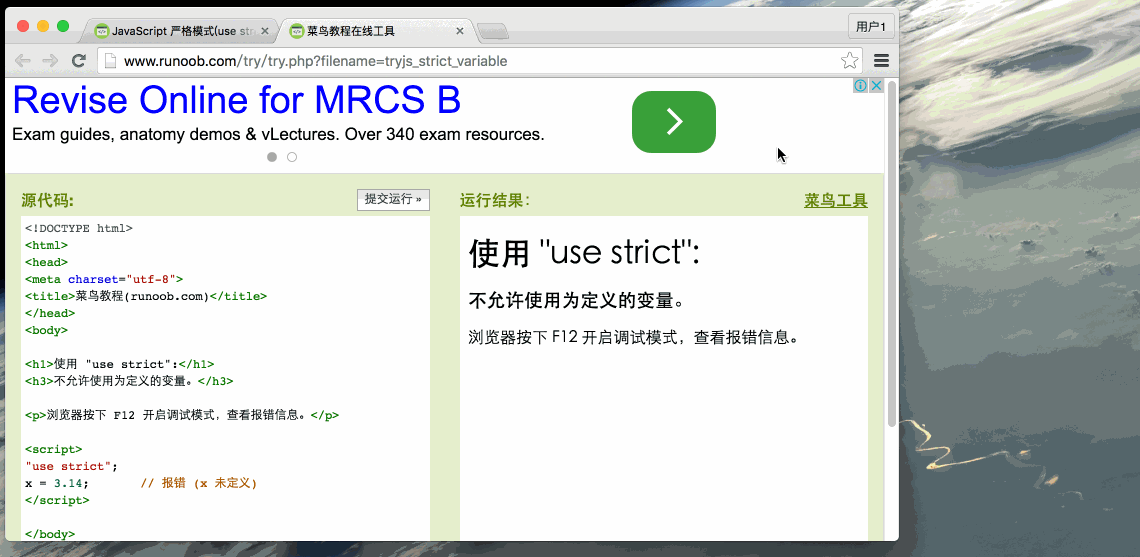2016

18JavaScript 严格模式（strict mode）即在严格的条件下运行。

## 使用 "use strict" 指令

"use strict" 指令在 JavaScript 1.8.5 (ECMAScript5) 中新增。

"use strict" 的目的是指定代码在严格条件下执行。支持严格模式的浏览器:Internet Explorer 10 +、 Firefox 4+ Chrome 13+、 Safari 5.1+、 Opera 12+。

## 严格模式声明

Gif 图演示如下：### 实例

"use strict";
x = 3.14;       // 报错 (x 未定义)

### 实例

"use strict";
myFunction();

function myFunction() {
y = 3.14;   // 报错 (y 未定义)
}

### 实例

x = 3.14;       // 不报错
myFunction();

function myFunction() {
"use strict";
y = 3.14;   // 报错 (y 未定义)
}

消除Javascript语法的一些不合理、不严谨之处，减少一些怪异行为;
• 消除代码运行的一些不安全之处，保证代码运行的安全；
• 提高编译器效率，增加运行速度；
• 为未来新版本的Javascript做好铺垫。

"严格模式"体现了Javascript更合理、更安全、更严谨的发展方向，包括IE 10在内的主流浏览器，都已经支持它，许多大项目已经开始全面拥抱它。

## 严格模式的限制

"use strict";
x = 3.14;                // 报错 (x 未定义)对象也是一个变量。

"use strict";
x = {p1:10, p2:20};      // 报错 (x 未定义)

"use strict";
var x = 3.14;
delete x;                // 报错

"use strict";
function x(p1, p2) {};
delete x;                // 报错

"use strict";
function x(p1, p1) {};   // 报错

"use strict";
var x = 010;             // 报错

"use strict";
var x = \010;            // 报错

"use strict";
var obj = {};
Object.defineProperty(obj, "x", {value:0, writable:false});

obj.x = 3.14;            // 报错

"use strict";
var obj = {get x() {return 0} };

obj.x = 3.14;            // 报错

"use strict";
delete Object.prototype; // 报错

"use strict";
var eval = 3.14;         // 报错

"use strict";
var arguments = 3.14;    // 报错

"use strict";
with (Math){x = cos(2)}; // 报错

"use strict";
eval ("var x = 2");

```function f(){
return !this;
}
// 返回false，因为"this"指向全局对象，"!this"就是false

function f(){
"use strict";
return !this;
}
// 返回true，因为严格模式下，this的值为undefined，所以"!this"为true。

```

```function f(){
"use strict";
this.a = 1;
};
f();// 报错，this未定义
```

## 保留关键字

• implements
• interface
• let
• package
• private
• protected
• public
• static
• yield
"use strict";
var public = 1500;      // 报错"use strict" 指令只运行出现在脚本或函数的开头。